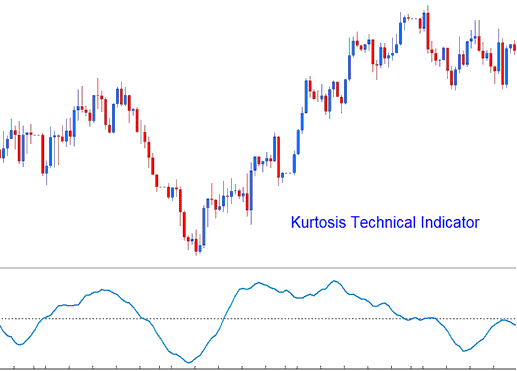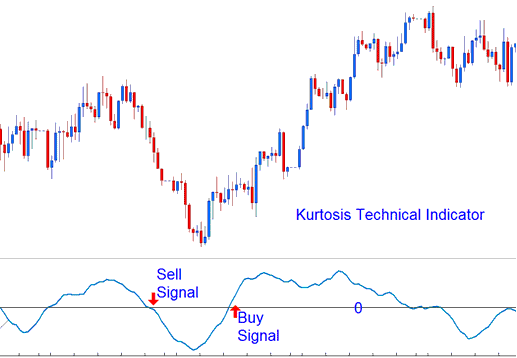# Kurtosis Stock Indices Technical Analysis and Kurtosis Stock Indices Signals

This indicator is used to help determine the sentiment of the stock index trading market.

It is calculated in 3 parts.

• Kurtosis portion

• Fast Kurtosis

• Fast/Slow Kurtosis

The Kurtosis portion is calculated using the formula:

K = n-periods momentum - n-periods momentum of 1 bar before (current stock index price bar’s momentum value minus the previous stock index price bar’s momentum value)

The Fast Kurtosis portion is calculated using the formula:

FK = n-periods moving average of K

The Fast/Slow Kurtosis portion is calculated using the formula:

FSK = n-periods moving average of FK## Stock Indices Technical Analysis and Generating Stock Indices Signals

Bullish Signal - A buy stock indices signal is generated when the indicator crosses above zero.

Bearish Signal - A sell stock indices signal is generated when the indicator crosses below zero.Technical Analysis